﻿ Notebook

## SIGNAL PROCESSING Laboratory #4

### Plotting signals in Python (time scale and amplitude resolution)

#### M. Kociński, P. Strumiłło, A. Borowska-Terka Medical Electronics Division Institute of Electronics

In :
import funkcje_do_lab4 as f4
f4.hide()

Out:
The raw code for this IPython notebook is by default hidden for easier reading. To toggle on/off the raw code, click here.

PURPOSE:

Plotting signals in Python.

1. Plotting sampled sinusoidal signals in a correct time scale:

In :
f4.fig(f4.p)

Out: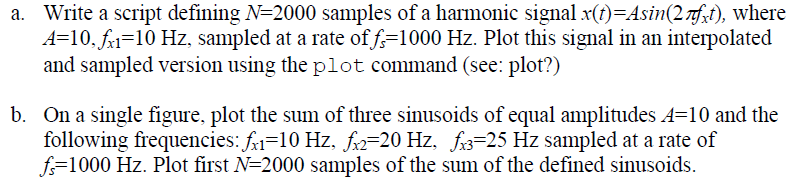EXPECTED RESULTS:

In :
%pylab inline
pylab.rcParams['figure.figsize'] = (14.0, 7.0)


Populating the interactive namespace from numpy and matplotlib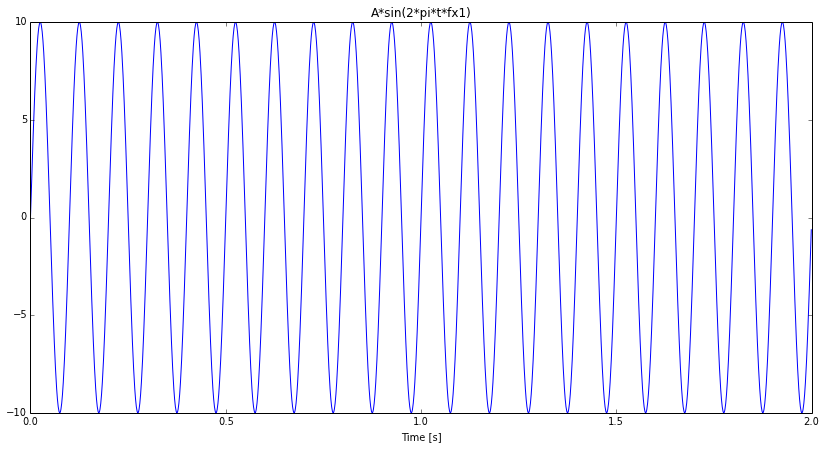Task b.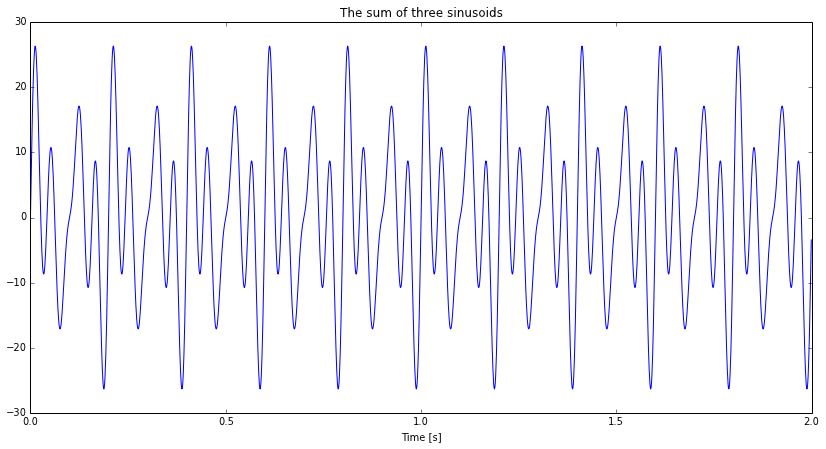2. Load ecg_mit.mat signal available in Signal files package from Signal Processing course webside.
Plot first N=2000 samples of this ECG signal in a correct time (in seconds) and amplitude (in mV) scale, given the sampling rate is fs=360 Hz and an 11-bit analog to digital (A/D) converter was applied to sample and code the ECG signal recorded in the voltage range of -5mV and +5mV.

EXPECTED RESULTS:

In :
f4.task_2()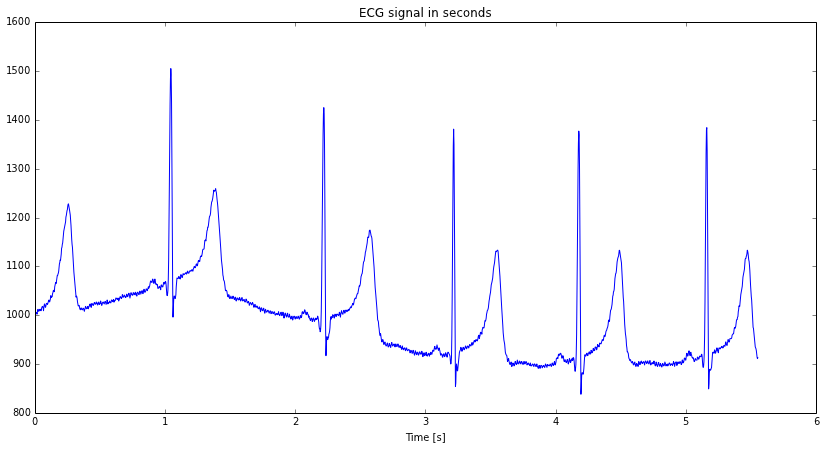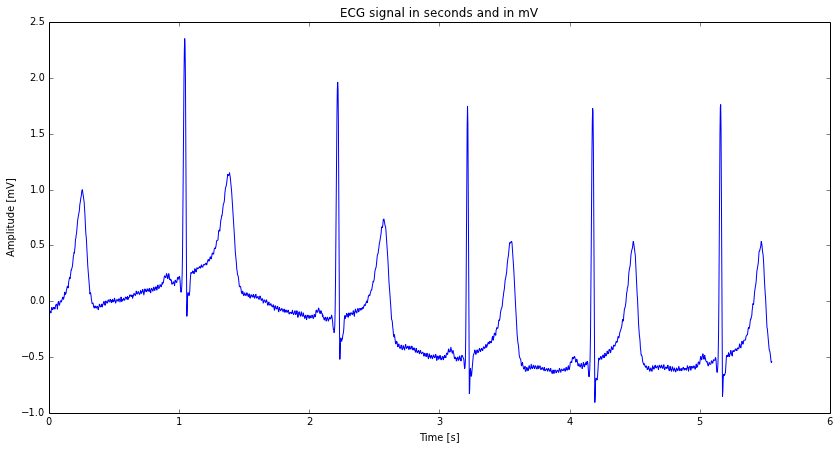In :



3. Write a function quantize_ecg(vec,b) that will simulate a lower number of bits of the A/D converter. The vec parameter is the vector containing the source 11-bit ECG signal and b is the parameter indicating by how many bits we decrease the resolution of an 11-bit ECG signal, e.g. if b=2 the function should return an ECG signal coded with 9 bits (i.e.11-b=9)

EXPECTED RESULTS:

In :
from scipy.io import loadmat
ecg = reshape(x, len(x))
ecg1 = ecg[:2000]

print 'Graph for b = 1:'
b = 1
new_ecg = f4.quantize_ecg(ecg1,b)
figure()
plot(new_ecg)
title('Number of bits coding one sample %d'%(11-b))
show()

print 'Graph for b = 2:'
b = 2
new_ecg = f4.quantize_ecg(ecg1,b)
figure()
plot(new_ecg)
title('Number of bits coding one sample %d'%(11-b))
show()

print 'Graph for b = 3:'
b = 3
new_ecg = f4.quantize_ecg(ecg1,b)
figure()
plot(new_ecg)
title('Number of bits coding one sample %d'%(11-b))
show()

print 'Graph for b = 4:'
b = 4
new_ecg = f4.quantize_ecg(ecg1,b)
figure()
plot(new_ecg)
title('Number of bits coding one sample %d'%(11-b))
show()

print 'Graph for b = 5:'
b = 5
new_ecg = f4.quantize_ecg(ecg1,b)
figure()
plot(new_ecg)
title('Number of bits coding one sample %d'%(11-b))
show()

print 'Graph for b = 6:'
b = 6
new_ecg = f4.quantize_ecg(ecg1,b)
figure()
plot(new_ecg)
title('Number of bits coding one sample %d'%(11-b))
show()

print 'Graph for b = 7:'
b = 7
new_ecg = f4.quantize_ecg(ecg1,b)
figure()
plot(new_ecg)
title('Number of bits coding one sample %d'%(11-b))
show()

Graph for b = 1: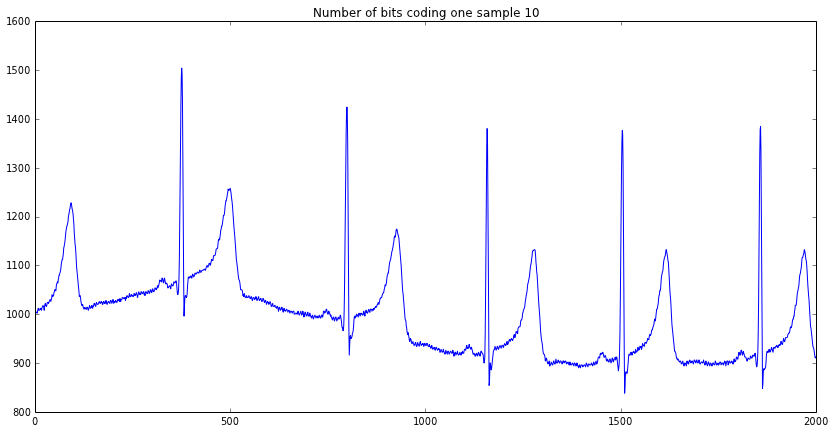Graph for b = 2: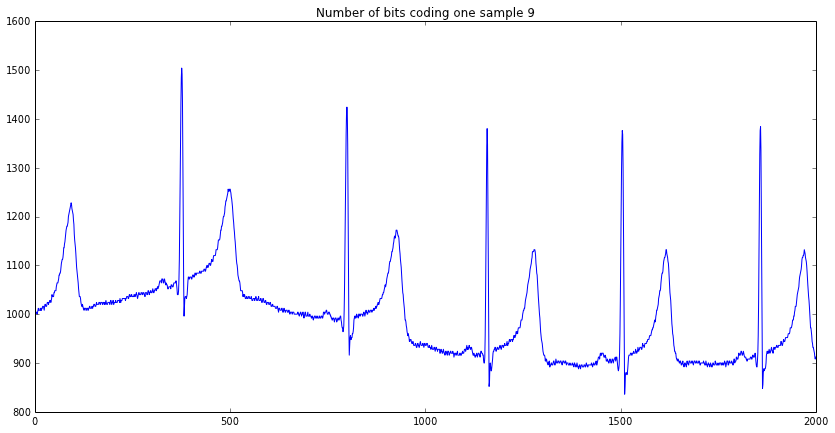Graph for b = 3: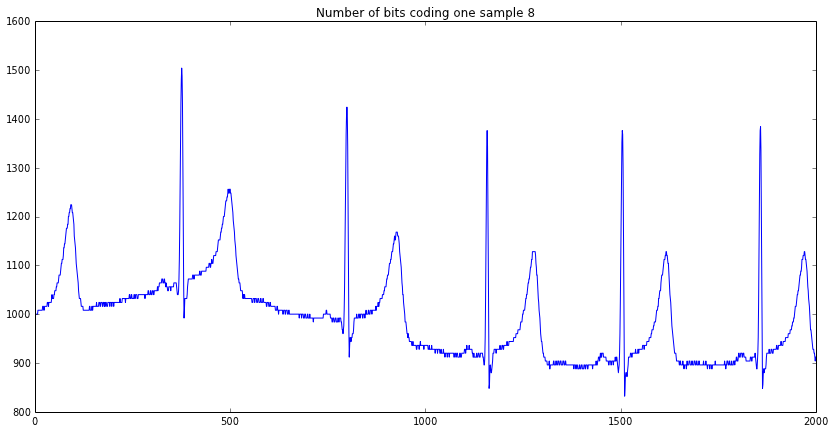Graph for b = 4: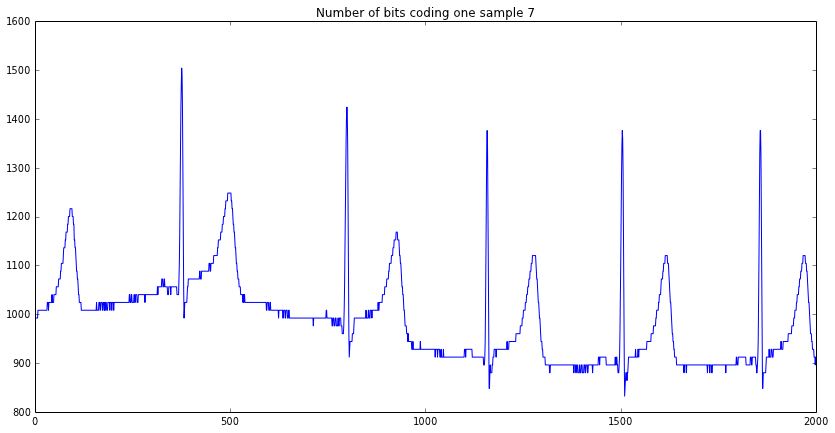Graph for b = 5: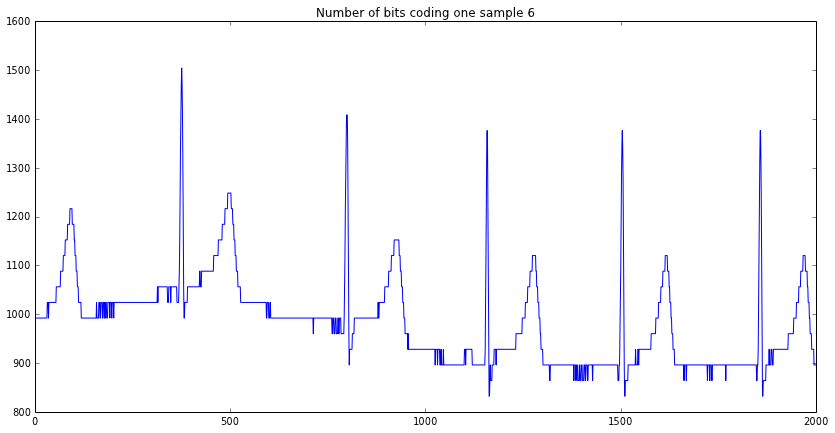Graph for b = 6: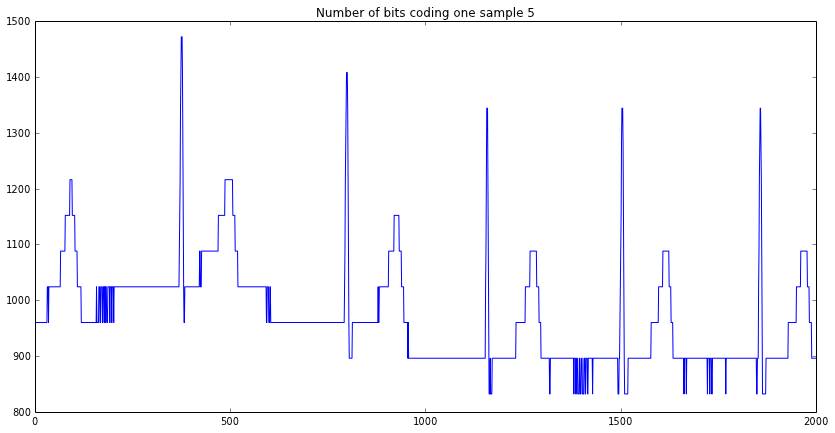Graph for b = 7: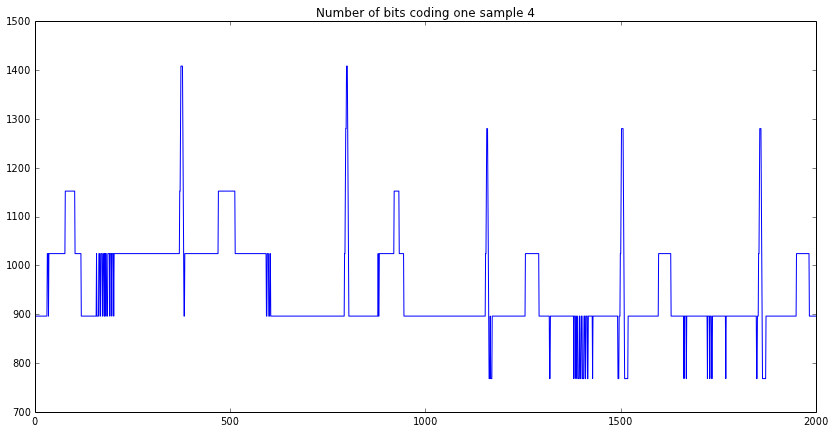In :